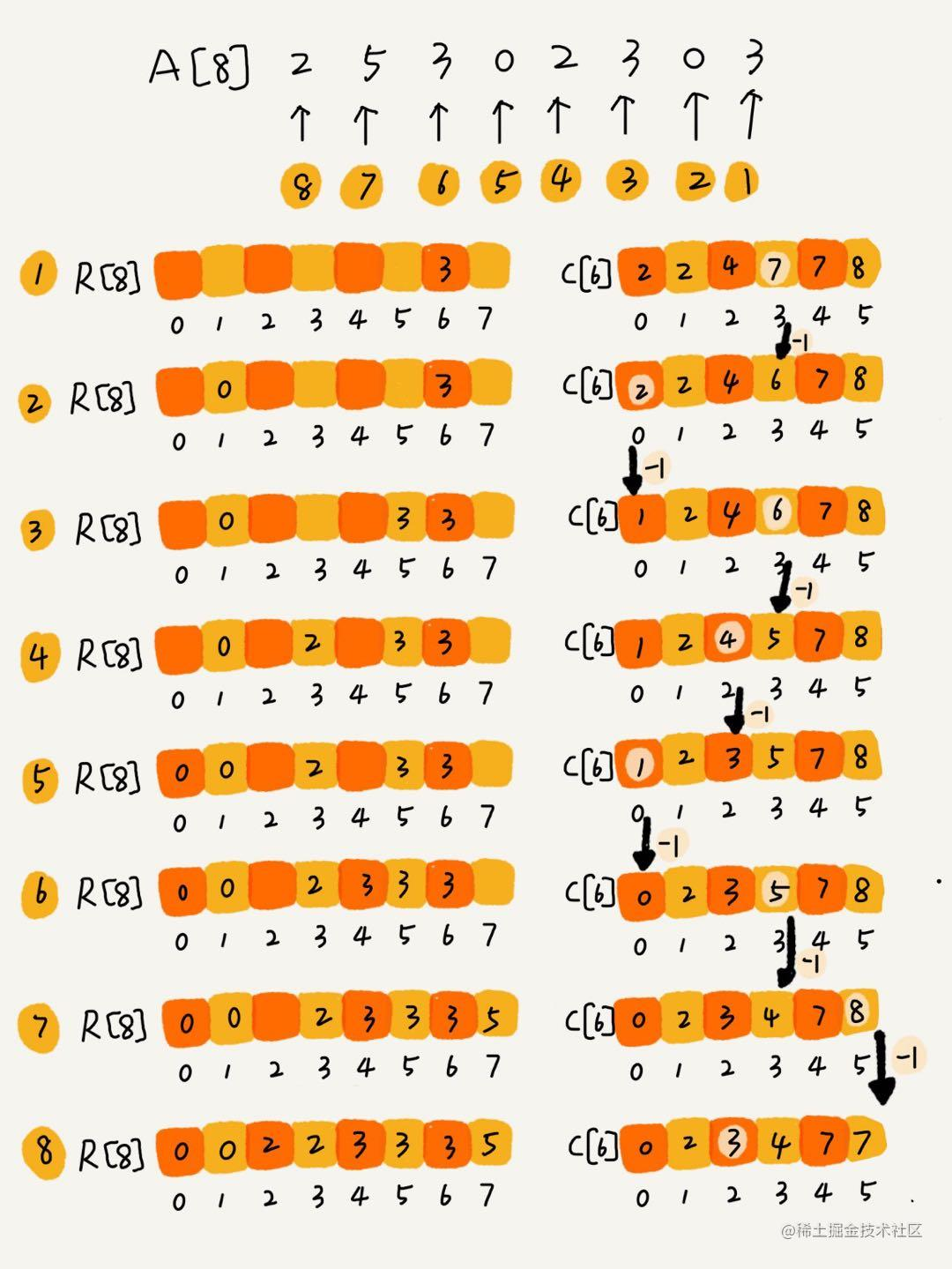# 排序算法总结

• O(n*n)：冒泡，插入，选择
• O(nlogn)：归并，快速
• O(n)：桶，计数，基数

### 冒泡排序

``````// 冒泡排序，a表示数组，n表示数组大小
public void bubbleSort(int[] a, int n) {
if (n <= 1) return;

for (int i = 0; i < n; ++i) {
// 提前退出冒泡循环的标志位
boolean flag = false;
for (int j = 0; j < n - i - 1; ++j) {
if (a[j] > a[j+1]) { // 交换
int tmp = a[j];
a[j] = a[j+1];
a[j+1] = tmp;
flag = true;  // 表示有数据交换
}
}
if (!flag) break;  // 没有数据交换，提前退出
}
}

### 插入排序

``````// 插入排序，a表示数组，n表示数组大小
public void insertionSort(int[] a, int n) {
if (n <= 1) return;

for (int i = 1; i < n; ++i) {
int value = a[i];
int j = i - 1;
// 查找插入的位置
for (; j >= 0; --j) {
if (a[j] > value) {
a[j+1] = a[j];  // 数据移动
} else {
break;
}
}
a[j+1] = value; // 插入数据
}
}

### 选择排序

``````public static void selectionSort(int[] arr){
for(int i = 0; i < arr.length - 1; i++){
//假设每次循环时，最小数的索引为i
int minIndex = i;     //每一个元素都和剩下的未排序的元素比较
for(int j = i + 1; j < arr.length; j++){
if(arr[j] < arr[minIndex]){
minIndex = j;//将最小数的索引保存
}
//经过一轮循环，就可以找出第一个最小值的索引，然后把最小值放到i的位置
int temp = arr[i];
arr[i] = arr[minIndex];
arr[j] = temp;
}
}

``````冒泡排序中数据的交换操作：
if (a[j] > a[j+1]) { // 交换
int tmp = a[j];
a[j] = a[j+1];
a[j+1] = tmp;
flag = true;
}

if (a[j] > value) {
a[j+1] = a[j];  // 数据移动
} else {
break;
}

### 归并排序

``````// 归并排序算法, A是数组，n表示数组大小
merge_sort(A, n) {
merge_sort_c(A, 0, n-1)
}

// 递归调用函数
merge_sort_c(A, p, r) {
// 递归终止条件
if p >= r  then return
// 取p到r之间的中间位置q
q = (p+r) / 2
// 分治递归
merge_sort_c(A, p, q)
merge_sort_c(A, q+1, r)
// 将A[p...q]和A[q+1...r]合并为A[p...r]
merge(A[p...r], A[p...q], A[q+1...r])
}

merge(A[p...r], A[p...q], A[q+1...r]) {
var i := p，j := q+1，k := 0 // 初始化变量i, j, k
var tmp := new array[0...r-p] // 申请一个大小跟A[p...r]一样的临时数组
while i<=q AND j<=r do {
if A[i] <= A[j] {
tmp[k++] = A[i++]
} else {
tmp[k++] = A[j++]
}
}
// 判断哪个子数组中有剩余的数据
var start := i，end := q
if j<=r then start := j, end:=r
// 将剩余的数据拷贝到临时数组tmp
while start <= end do {
tmp[k++] = A[start++]
}
// 将tmp中的数组拷贝回A[p...r]
for i:=0 to r-p do {
A[p+i] = tmp[i]
}
}

### 快速排序

``````const quickSort = (array) => {
const sort = (arr, left = 0, right = arr.length - 1) => {
if (left >= right) {
//如果左边的索引大于等于右边的索引说明整理完毕
return
}
let i = left
let j = right
const baseVal = arr[j] // 取无序数组最后一个数为基准值
while (i < j) {
//把所有比基准值小的数放在左边大的数放在右边
while (i < j && arr[i] <= baseVal) {
//找到一个比基准值大的数交换
i++
}
arr[j] = arr[i] // 将较大的值放在右边如果没有比基准值大的数就是将自己赋值给自己（i 等于 j）
while (j > i && arr[j] >= baseVal) {
//找到一个比基准值小的数交换
j--
}
arr[i] = arr[j] // 将较小的值放在左边如果没有找到比基准值小的数就是将自己赋值给自己（i 等于 j）
}
arr[j] = baseVal // 将基准值放至中央位置完成一次循环（这时候 j 等于 i ）
sort(arr, left, j-1) // 将左边的无序数组重复上面的操作
sort(arr, j+1, right) // 将右边的无序数组重复上面的操作 }
const newArr = array.concat() // 为了保证这个函数是纯函数拷贝一次数组
sort(newArr)
return newArr
}
}

### 线性排序算法

• 线性排序算法包括桶排序、计数排序、基数排序
• 线性排序算法的时间复杂度为O(n)。
• 对排序数据的要求很苛刻，重点掌握此3种排序算法的适用场景。

### 桶排序

• 将要排序的数据分到几个有序的桶里，每个桶里的数据再单独进行快速排序。
• 桶内排完序之后，再把每个桶里的数据按照顺序依次取出，组成的序列就是有序的了。

• 要排序的数据需要很容易就能划分成m个桶，并且桶与桶之间有着天然的大小顺序。

• 数据在各个桶之间分布是均匀的。

• 桶排序比较适合用在外部排序中。
• 外部排序就是数据存储在外部磁盘且数据量大，但内存有限无法将整个数据全部加载到内存中。

• 需求描述： 有10GB的订单数据，需按订单金额（假设金额都是正整数）进行排序 但内存有限，仅几百MB。
• 解决思路： 扫描一遍文件，看订单金额所处数据范围，比如1元-10万元，那么就分100个桶。 第一个桶存储金额1-1000元之内的订单，第二个桶存1001-2000元之内的订单，依次类推。 每个桶对应一个文件，并按照金额范围的大小顺序编号命名（00，01，02，…，99）。 将100个小文件依次放入内存并用快排排序。 所有文件排好序后，只需按照文件编号从小到大依次读取每个小文件并写到大文件中即可。
• 注意点：若单个文件无法全部载入内存，则针对该文件继续按照前面的思路进行处理即可。

### 计数排序

• 计数其实就是桶排序的一种特殊情况。

• 当要排序的n个数据所处范围并不大时，比如最大值为k，则分成k个桶。

• 每个桶内的数据值都是相同的，就省掉了桶内排序的时间。

• 只能用在数据范围不大的场景中，若数据范围k比要排序的数据n大很多，就不适合用计数排序。
• 计数排序只能给非负整数排序，其他类型需要在不改变相对大小情况下，转换为非负整数。
• 比如如果考试成绩精确到小数后一位，就需要将所有分数乘以10，转换为整数。

•  假设只有8个考生分数在0-5分之间，成绩存于数组A = [2，5，3，0，2，3，0，3]。

• 使用大小为6的数组C表示桶，下标对应分数，即0，1，2，3，4，5。 C存储的是考生人数，只需遍历一边考生分数，就可以得到C = [2，0，2，3，0，1]。

• 对C数组顺序求和则C=[2，2，4，7，7，8]，c[k]存储的是小于等于分数k的考生个数。

• 数组R = [0，0，2，2，3，3，3，5]存储考生名次。那么如何得到R的呢？ 从后到前依次扫描数组A，比如扫描到3时，可以从数组C中取出下标为3的值7，也就是说，到目前为止，包括自己在内，分数小于等于3的考生有7个，也就是说3是数组R的第7个元素（也就是数组R中下标为6的位置）。当3放入数组R后，小于等于3的元素就剩下6个了，相应的C要减1变成6。 以此类推，当扫描到第二个分数为3的考生时，就会把它放入数组R中第6个元素的位置（也就是下标为5的位置）。当扫描完数组A后，数组R内的数据就是按照分数从小到大排列的了。（这么做是为了保证稳定排序）

•### 基数排序

• 如果只比较两个手机号码a，b的大小，如果在前面几位中a已经比b大了，那后面几位就不用看了。

• 比较多个手机号码，借助稳定排序算法的思想，可以先按照最后一位来排序手机号码，然后再按照倒数第二位来重新排序，以此类推。注意，这里按照每位来排序的算法要是稳定的，否则这个实现思路就是不正确的。

• 经过11次排序后，手机号码就变为有序的了。

• 每次排序有序数据范围较小，可以使用桶排序或计数排序来完成。

• 要求数据可以分割独立的“位”来比较。
• 位之间由递进关系，如果a数据的高位比b数据大，那么剩下的低位就不用比较了。
• 每一位的数据范围不能太大，要可以用线性排序，否则基数排序的时间复杂度无法做到O(n)。

### 如何实现一个通用的，高性能的排序函数

1.  三数取中法 我们从区间的首、尾、中间，分别取出一个数，然后对比大小，取这 3 个数的中间值作为分区点。这样每间隔某个固定的长度，取数据出来比较，将中间值作为分区点的分区算法，肯定要比单纯取某一个数据更好。但是，如果要排序的数组比较大，那“三数取中”可能就不够了，可能要“五数取中”或者“十数取中”。
2. 随机法 随机法就是每次从要排序的区间中，随机选择一个元素作为分区点。这种方法并不能保证每次分区点都选的比较好，但是从概率的角度来看，也不大可能会出现每次分区点都选得很差的情况，所以平均情况下，这样选的分区点是比较好的。时间复杂度退化为最糟糕的 O(n2) 的情况，出现的可能性不大。

**在小规模数据面前，O(n*n) 时间复杂度的算法并不一定比 O(nlogn) 的算法执行时间长。**因为在大 O 复杂度表示法中，我们会省略低阶、系数和常数，也就是说，O(nlogn) 在没有省略低阶、系数、常数之前可能是 O(knlogn + c)，而且 k 和 c 有可能还是一个比较大的数。